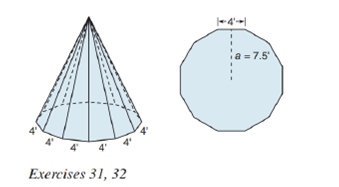Chapter 9.2, Problem 31EElementary Geometry For College St...

7th Edition
Alexander + 2 others
ISBN: 9781337614085

Solutions

Chapter
SectionElementary Geometry For College St...

7th Edition
Alexander + 2 others
ISBN: 9781337614085
Textbook Problem

A teepee is constructed by using 12 poles. The construction leads to a regular pyramid with a dodecagon (12 sides) for the base. With the base as shown, and knowing that the height of the teepee is 15 f t , find its volume.To determine

To find:

The volume of the pyramid.

Explanation

Given:

A teepee in shape of a regular pyramid with a dodecagon (12 sides) for the base as below,

and the height of the teepee is 15ft and side of the base as 4ft and apothem a=7.5ft.

Properties Used:

A pyramid is made by connecting a base to an apex. The base is flat with straight edges, no curves, hence, a polygon and all other faces are triangles.

A regular pyramid is a pyramid whose base is a regular polygon and whose lateral edges are all congruent.

The lateral area L of a regular pyramid with slant height of length l and perimeter P of the base is given by

L=12lP.

The total surface area T of a pyramid with lateral area L and base area B is given by

T=L+B.

According to the Pythagorean theorem, in a right-angled triangle

hypotenuse2=base2+perpendicular2.

In a regular pyramid, the lengths of the apothem a of the base, the altitude h, and the slant height l satisfy the Pythagorean Theorem; that is, l2=a2+h2.

The perimeter of the square is four times the side

Still sussing out bartleby?

Check out a sample textbook solution.

See a sample solution

The Solution to Your Study Problems

Bartleby provides explanations to thousands of textbook problems written by our experts, many with advanced degrees!

Get Started

Draw, in standard position, the angle whose measure is given. 22. 3 rad

Single Variable Calculus: Early Transcendentals, Volume I

In problems 37-48, compute and simplify so that only positive exponents remain. 38.

Mathematical Applications for the Management, Life, and Social Sciences

In Exercises 35-38, find an equation of the line that has slope m and y-intercept b. 36. m = 2; b = 1

Applied Calculus for the Managerial, Life, and Social Sciences: A Brief Approach